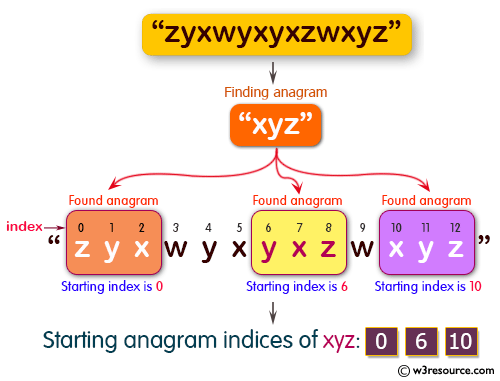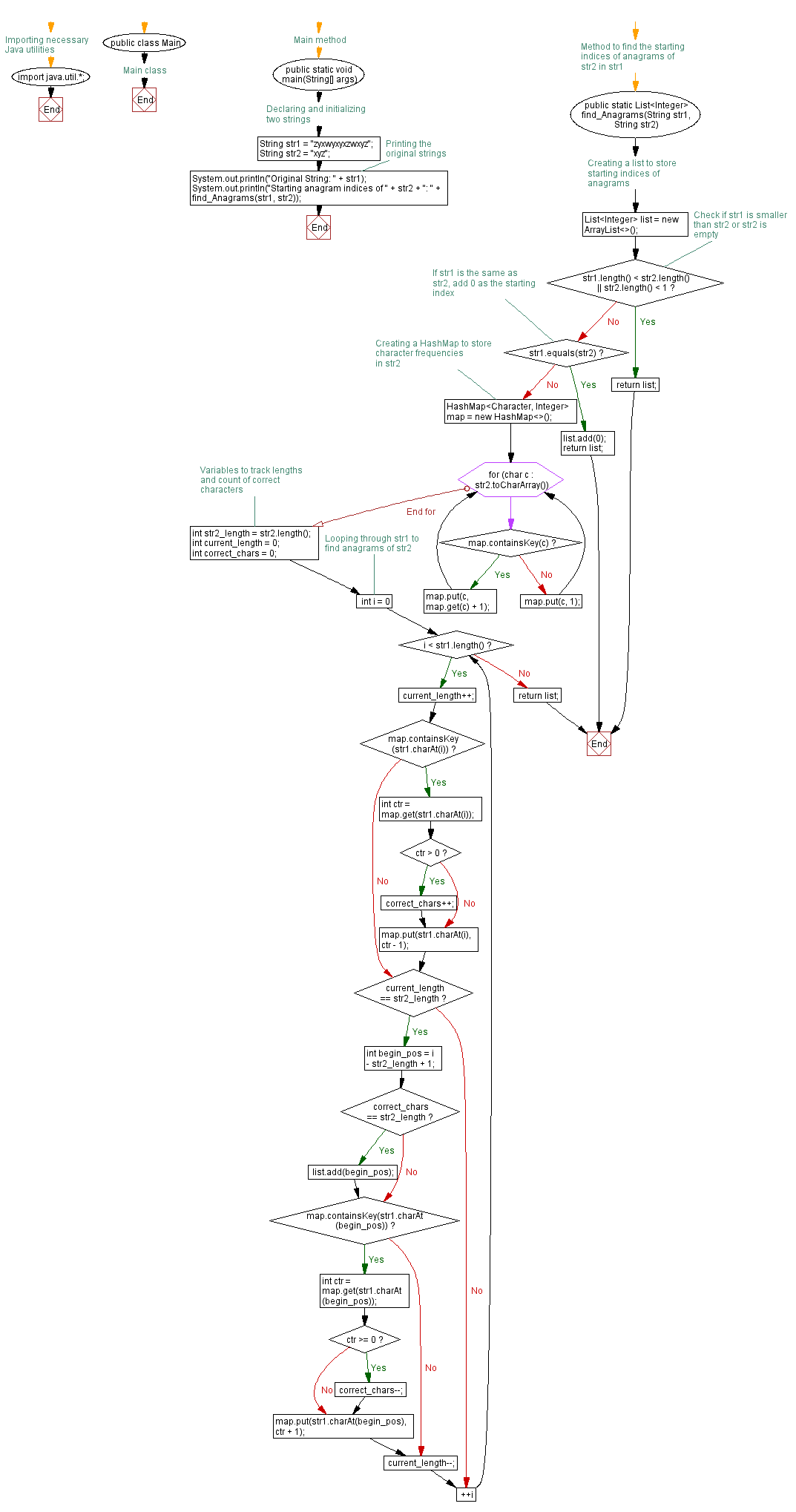﻿ Java programming exercises: Find all the start indices of a given string's anagrams in another specified string - w3resource# Java Exercises: Find all the start indices of a given string's anagrams in another specified string

## Java Basic: Exercise-188 with Solution

Write a Java program to find all the start indices of a given string's anagrams in another given string.

Pictorial Presentation:Sample Solution:

Java Code:

``````import java.util.*;
public class Solution {
public static void main(String[] args) {
String str1 = "zyxwyxyxzwxyz";
String str2 = "xyz";
System.out.println("Original String: " + str1);
System.out.println("Starting anagram indices of " + str2 + ": " + find_Anagrams(str1, str2));
}

public static List < Integer > find_Anagrams(String str1, String str2) {
List < Integer > list = new ArrayList < Integer > ();
if (str1.length() < str2.length() || str2.length() < 1) {
return list;
}
if (str1.equals(str2)) {
return list;
}

HashMap < Character, Integer > map = new HashMap < > ();
for (char c: str2.toCharArray()) {
if (map.containsKey(c)) {
map.put(c, map.get(c) + 1);
} else {
map.put(c, 1);
}
}
int str2_length = str2.length();
int current_length = 0;
int correct_chars = 0;

for (int i = 0; i < str1.length(); ++i) {
current_length++;
if (map.containsKey(str1.charAt(i))) {
int ctr = map.get(str1.charAt(i));
if (ctr > 0) {
correct_chars++;
}
map.put(str1.charAt(i), ctr - 1);
}

if (current_length == str2_length) {
int begin_pos = i - str2_length + 1;
if (correct_chars == str2_length) {
}
if (map.containsKey(str1.charAt(begin_pos))) {
int ctr = map.get(str1.charAt(begin_pos));
if (ctr >= 0) {
correct_chars--;
}
map.put(str1.charAt(begin_pos), ctr + 1);
}
current_length--;
}
}
return list;
}
}
```
```

Sample Output:

```Original String: zyxwyxyxzwxyz
Starting anagram indices of xyz: [0, 6, 10]
```

Flowchart:Java Code Editor:

Company:

What is the difficulty level of this exercise?

﻿

## Java: Tips of the Day

Array vs ArrayLists:

The main difference between these two is that an Array is of fixed size so once you have created an Array you cannot change it but the ArrayList is not of fixed size. You can create instances of ArrayLists without specifying its size. So if you create such instances of an ArrayList without specifying its size Java will create an instance of an ArrayList of default size.

Once an ArrayList is full it re-sizes itself. In fact, an ArrayList is internally supported by an array. So when an ArrayList is resized it will slow down its performance a bit as the contents of the old Array must be copied to a new Array.

At the same time, it's compulsory to specify the size of an Array directly or indirectly while creating it. And also Arrays can store both primitives and objects while ArrayLists only can store objects.

Ref: https://bit.ly/3o8L2KH